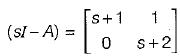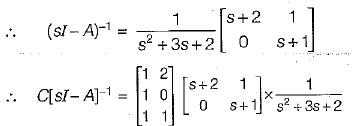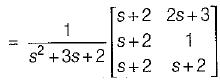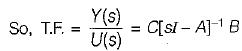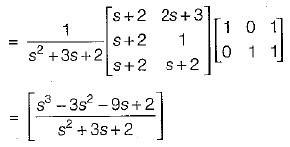Courses

# Test: State Variable Analysis

## 10 Questions MCQ Test Control Systems | Test: State Variable Analysis

Description
This mock test of Test: State Variable Analysis for Electrical Engineering (EE) helps you for every Electrical Engineering (EE) entrance exam. This contains 10 Multiple Choice Questions for Electrical Engineering (EE) Test: State Variable Analysis (mcq) to study with solutions a complete question bank. The solved questions answers in this Test: State Variable Analysis quiz give you a good mix of easy questions and tough questions. Electrical Engineering (EE) students definitely take this Test: State Variable Analysis exercise for a better result in the exam. You can find other Test: State Variable Analysis extra questions, long questions & short questions for Electrical Engineering (EE) on EduRev as well by searching above.
QUESTION: 1

Solution:
QUESTION: 2

Solution:
QUESTION: 3

### For a linear time invariant system, an optimal controller can be designed if

Solution:

An optimal controller for an LTI system can be designed provided the system is both controllable and observable

QUESTION: 4

The eigen values of linear system are the location of

Solution:

Eigen value are given by | sI - A | = 0, which is the location of poles.

QUESTION: 5

If the system matrix of a linear time invariant continuous system is given by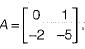Its characteristic equation will be given by

Solution:

Characteristic equation is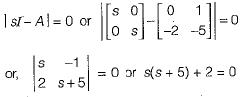or, s2 + 5s + 2 = 0

QUESTION: 6

​The state-variable description of a linear autonomous system is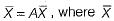is a two-dimensional state vector and A is a matrix given by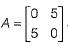The poles of the system are located at

Solution:

Poles of given system are given by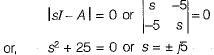QUESTION: 7

Assertion (A): The eigen values of a linear continuous-data time invariant system controls the stability of the system.
Reason (R): The roots of the characteristic equation are the same as the eigen values of system matrix A of the state equations.

Solution:
QUESTION: 8

The vector matrix differential equation of a system is given by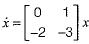The state transition matrix of the system is

Solution:

We have: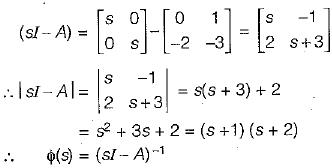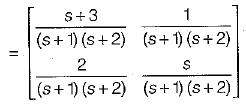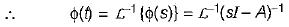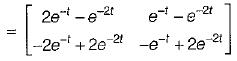QUESTION: 9

The system matrix of a continous time system is given by: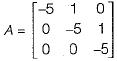​The characteristic equation is

Solution:

The characteristic equation | sI - A | = 0.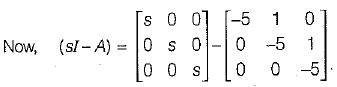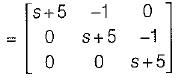Now, | sI - A | = 0
or, (s + 5) [(s + 5)2 + 0] + 1 (0 - 0) + 0 = 0
or, (s + 5)3 = 0 or s3 + 15s2 + 75s + 125 = 0

QUESTION: 10

A system is described by the following equations: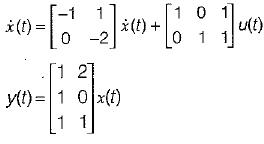The transfer function of the system is

Solution:

Transfer function is given by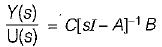Given,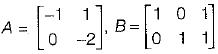and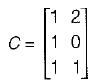Now,